# Easy Bode Plots ExplainedThe frequency range required in frequency response is often so wide that it is inconvenient to use a linear scale for the frequency axis. Also, there is a more systematic way of locating the important features of the magnitude and phase plots of the transfer

Contents

For these reasons, it has become standard practice to use a logarithmic scale for the frequency axis and a linear scale in each of the separate plots of magnitude and phase.

## Bode Plots

Such semilogarithmic plots of the transfer function—known as Bode plots—have become the industry standard.

Bode plots are semilog plots of the magnitude (in decibels) and phase (in degrees) of a transfer function versus frequency.

Bode plots contain the same information as the nonlogarithmic plots discussed in the previous section, but they are much easier to construct, as we shall see shortly.

The transfer function can be written as

Taking the natural logarithm of both sides,

Thus, the real part of ln H is a function of the magnitude while the imaginary part is the phase. In a Bode magnitude plot, the gain

is plotted in decibels (dB) versus frequency. Table.(1) provides a few values of H with the corresponding values in decibels.

-table 1 ( Specific gains and their decibel values.

In a Bode phase plot, φ is plotted in degrees versus frequency. Both magnitude and phase plots are made on semilog graph paper.

A transfer function in the form of Equation.(2) may be written in terms of factors that have real and imaginary parts. One such representation might be

which is obtained by dividing out the poles and zeros in H(ω). The representation of H(ω) as in Equation.(4) is called the standard form. In this particular case, H(ω) has seven different factors that can appear in various combinations in a transfer function. These are:

1. A gain K
2. A pole (jω)−1 or zero (jω) at the origin
3. A simple pole 1/(1 + jω/p1) or zero (1 + jω/z1)
4. A quadratic pole 1/[1 + j2ζ2ω/ωn + (jω/ωn)2] or zero [1 + j2ζ1ω/ωk + (jω/ωk)2]

In constructing a Bode plot, we plot each factor separately and then combine them graphically. The factors can be considered one at a time and then combined additively because of the logarithms involved.

It is this mathematical convenience of the logarithm that makes Bode plots a powerful engineering tool.

We will now make straight-line plots of the factors listed above. We
shall find that these straight-line plots known as Bode plots approximate the actual plots to a surprising degree of accuracy.

Constant term: For the gain K, the magnitude is 20 log10K and the
phase is 0◦; both are constant with frequency. Thus the magnitude and phase plots of the gain are shown in Figure.(1). If K is negative, the magnitude remains 20 log10|K| but the phase is ±180◦.

Pole/zero at the origin: For the zero (jω ) at the origin, the magnitude is 20 log10 ω and the phase is 90 ◦. These are plotted in Figure.(2), where we notice that the slope of the magnitude plot is 20 dB/decade, while the phase is constant with frequency.Figure 2. Bode plot for a zero (jω) at the origin: (a) magnitude plot, (b) phase plot.

A decade is an interval between two frequencies with a ratio of 10; e.g., between ω0 and 10ω0, or between 10 and 100 Hz. Thus, 20 dB/decade means that the magnitude changes 20 dB whenever the frequency changes tenfold or one decade.

The Bode plots for the pole (jω)−1 are similar except that the slope of the magnitude plot is −20 dB/decade while the phase is −90◦. In general, for (jω)N, where N is an integer, the magnitude plot will have a slope of 20N dB/decade, while the phase is 90N degrees.

Simple pole/zero: For the simple zero (1 + jω/z1), the magnitude is 20 log10 |1 + jω/z1| and the phase is tan−1ω/z1. We notice that

showing that we can approximate the magnitude as zero (a straight line with zero slope) for small values of ω and by a straight line with slope 20 dB/decade for large values of ω.

The frequency ω = z1 where the two asymptotic lines meet is called the corner frequency or break frequency.

Thus the approximate magnitude plot is shown in Figure.(3a), where the actual plot is also shown.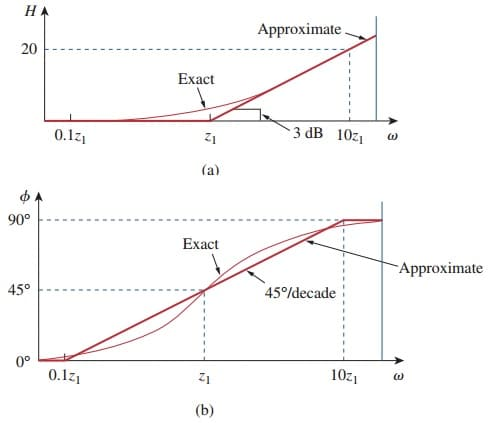Figure 3. Bode plots of zero (1 + jω/z1 ): (a) magnitude plot, (b) phase plot

Notice that the approximate plot is close to the actual plot except at the break frequency, where ω = z1 and the deviation is 20 log10|(1 + j1)| = 20 log10 √2 = 3 dB.

The phase tan−1(ω/z1) can be expressed as

As a straight-line approximation, we let φ ≅ 0 for ω z1/10, φ ≅ 45◦ for ω = z1, and φ ≅ 90◦ for ω ≥ 10z1. As shown in Figure.(3b) along with the actual plot, the straight-line plot has a slope of 45◦ per decade.

The Bode plots for the pole 1/(1 + jω/p1) are similar to those in Figure.(3) except that the corner frequency is at ω = p1, the magnitude has a slope of −20 dB/decade, and the phase has a slope of −45 ◦ per decade.

Quadratic pole/zero: The magnitude of the quadratic pole 1 /[1 + j2 ζ2ω /ωn + (jω/ωn)2] is −20 log10 |1 + j2ζ2ω/ωn + (jω/ωn)2| and the phase is − tan−1(2ζ2ω/ωn)/(1 − ω/ωn2). But

and

Thus, the amplitude plot consists of two straight asymptotic lines: one with zero slope for ω < ωn and the other with slope -40dB/decade for ω > ωn, with ωn as the corner frequency. Figure.(4a) shows the approximate and actual amplitude plots.

Note that the actual plot depends on the damping factor ζ2 as well as the corner frequency ωn.

The significant peaking in the neighborhood of the corner frequency should be added to the straight-line approximation if a high level of accuracy is desired.

However, we will use the straight-line approximation for the sake of simplicity.

The phase can be expressed as

The phase plot is a straight line with a slope of 90◦ per decade starting at ωn/10 and ending at 10ωn, as shown in Figure.(4b).

We see again that the difference between the actual plot and the straight-line plot is due to the damping factor.

Notice that the straight-line approximations for both magnitude and phase plots for the quadratic pole are the same as those for a double pole, i.e. (1 + jω/ωn)−2.

We should expect this because the double pole (1 + jω/ωn)−2 equals the quadratic pole 1/[1 + j2ζ2ω/ωn + (jω/ωn)2] when ζ2 = 1.

Thus, the quadratic pole can be treated as a double pole as far as straight-line approximation is concerned.

For the quadratic zero [1 +j2ζ1ω/ωk +(jω/ωk)2], the plots in Figure.(4) are inverted because the magnitude plot has a slope of 40 dB/decade while the phase plot has a slope of 90◦ per decade. Table.(2) presents a summary of Bode plots for the seven factors.

To sketch the Bode plots for a function H(ω) in the form of Equation.(4), for example, we first record the corner frequencies on the semilog graph paper, sketch the factors one at a time as discussed above, and then combine additively the graphs of the factors.

The combined graph is often drawn from left to right, changing slopes appropriately each time a corner frequency is encountered. The following examples illustrate this procedure.

-table 2 ( Summary of Bode straight-line magnitude and phase plots.

## Bode Plots Examples

Let’s review the bode plots examples below:

### Bode Plots Example 1

Construct the Bode plots for the transfer function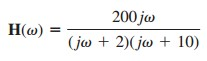Solution:
We first put H(ω) in the standard form by dividing out the poles and zeros. Thus,Hence the magnitude and phase are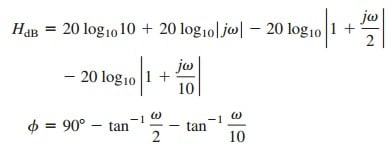We notice that there are two corner frequencies at ω = 2, 10. For both the magnitude and phase plots, we sketch each term as shown by the dotted lines in Figure.(5). We add them up graphically to obtain the overall plots shown by the solid curves.

### Bode Plots Example 2

Obtain the Bode plots forSolution:
Putting H (ω ) in the standard form, we get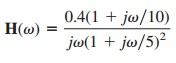From this, we obtain the magnitude and phase as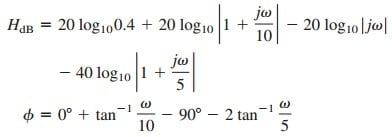There are two corner frequencies at ω = 5, 10 rad/s. For the pole with corner frequency at ω = 5, the slope of the magnitude plot is −40 dB/decade and that of the phase plot is −90◦ per decade due to the power of 2.

The magnitude and the phase plots for the individual terms (in dotted lines) and the entire H(jω) (in solid lines) are in Figure.(6).

### Bode Plots Example 3

Draw the Bode plots for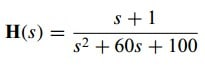Solution:

We express H (s ) as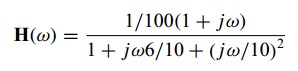For the quadratic pole, ωn = 10 rad/s, which serves as the corner frequency. The magnitude and phase areFigure.(7) shows the Bode plots. Notice that the quadratic pole is treated as a repeated pole at ωk, that is, (1 + jω/ωk)2, which is an approximation.

### Bode Plots Example 4

Given the Bode plot in Figure.(8), obtain the transfer function H(ω).

Solution:
To obtain H (ω ) from the Bode plot, we keep in mind that a zero always causes an upward turn at a corner frequency, while a pole causes a downward turn.

We notice from Figure.(8) that there is a zero j ω at the origin which should have intersected the frequency axis at ω = 1. This is indicated by the straight line with slope +20 dB/decade.

The fact that this straight line is shifted by 40 dB indicates that there is a 40-dB gain; that is,orIn addition to the zero j ω at the origin, we notice that there are three factors with corner frequencies at ω = 1 , 5, and 20 rad/s. Thus, we have:

1. A pole at p = 1 with slope −20 dB/decade to cause a downward turn and counteract the pole at the origin. The pole at
z = 1 is determined as 1 /(1 + jω/1).
2. Another pole at p = 5 with slope −20 dB/decade causing a
downward turn. The pole is 1/(1 + jω/5).
3. A third pole at p = 20 with slope −20 dB/decade causing a
further downward turn. The pole is 1/(1 + jω/20).

Putting all these together gives the corresponding transfer function asor